Zaynab Awofeso — Published On August 30, 2022 and Last Modified On August 31st, 2022

This article was published as a part of the Data Science Blogathon.

## IntroductionAs Josh Wills once said, “A Data Scientist is a person who is better at statistics than any programmer and better at programming than any statistician“.

Statistics is a fundamental tool when dealing with data and its analysis in Data Science. It provides tools and methods that assist a Data Scientist derive insights and interpret vast amounts of data. It is not enough to master Data Science tools and languages. You should also have a strong understanding of certain core statistical concepts and basics. Keeping this in mind, here is a list of 40 most frequently asked Statistics Data Science Interview Questions and Answers. It will help you refresh your memory of key aspects of statistics and help you prepare for job interviews encompassing Data Science and Machine Learning.

With that said, let’s get into it!

## Statistics Interview Questions and Answers

1. What is the difference between a population and a sample?

📍 A population represents the entirety of all items that are being studied.

📍 A sample is a finite subset of the population that is selected to represent the entire group. A sample is usually selected because the population is too large or costly to study in its entirety.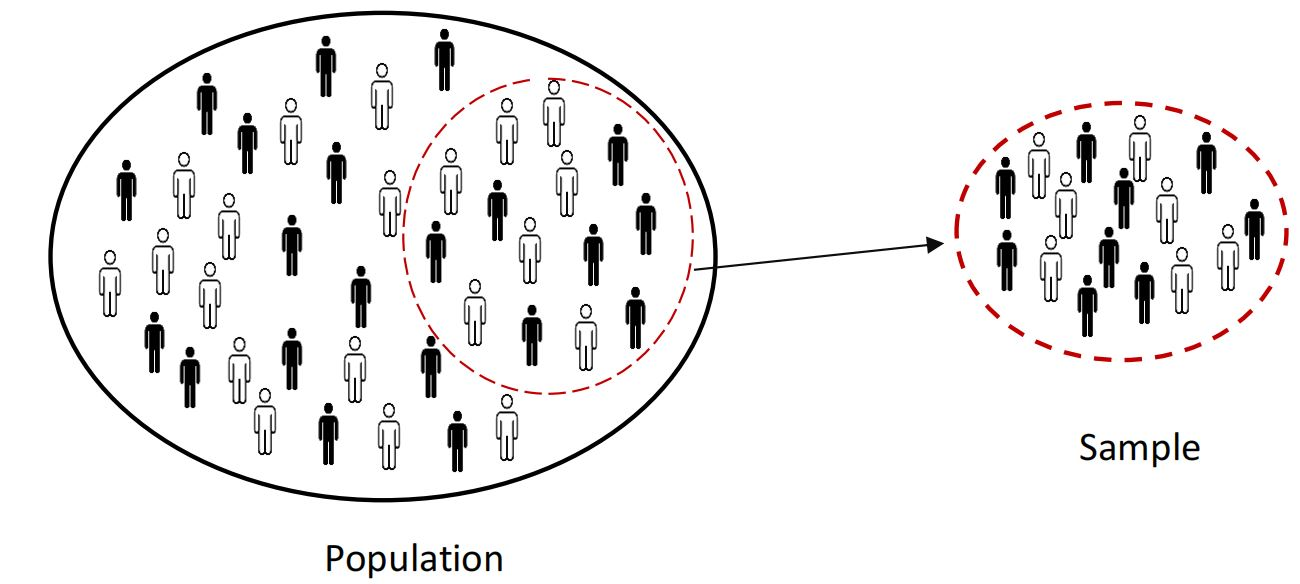An example of population data is a census, and a good example of a sample is a survey.

2. What is the difference between inferential and descriptive statistics?

📍 Descriptive statistics describes some sample or population.

📍Inferential statistics attempts to infer from some sample to the larger population.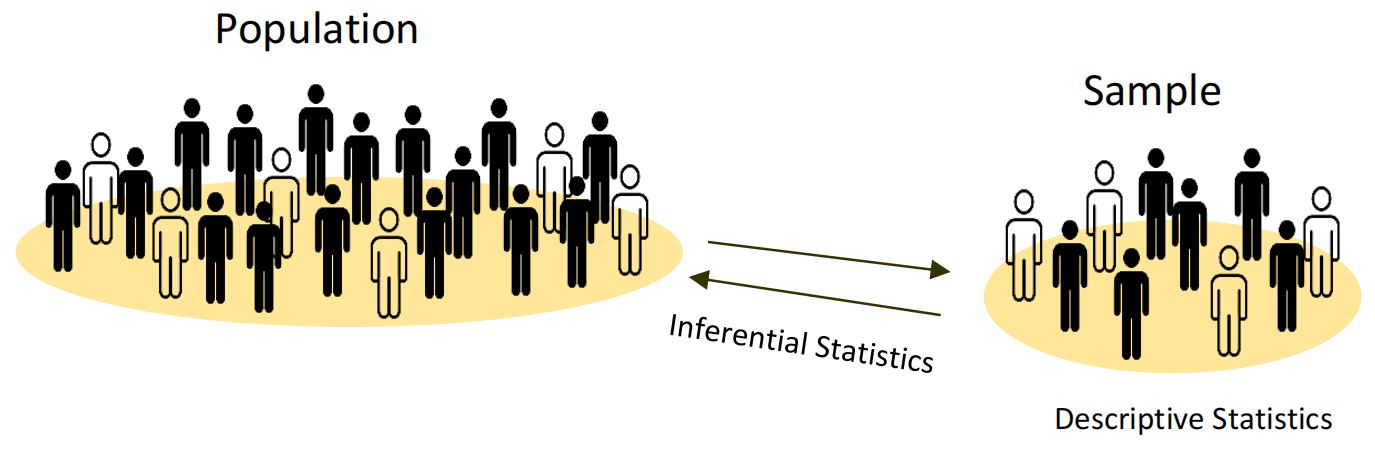3. What are quantitative and qualitative data?

📍 Quantitative data are measures of values or counts and are expressed as numbers. Quantitative data refers to numerical data (e.g. how many, how much, or how often).

📍 Qualitative data are measures of ‘types’ and may be represented by a name, symbol, or a number code. Qualitative data is also known as categorical data.

4. What is the meaning of standard deviation?

Standard deviation is a statistic that measures the dispersion of a dataset relative to its mean. It is the average amount of variability in your dataset. It tells you, on average, how far each value lies from the mean.

A high standard deviation means that values are generally far from the mean, while a low standard deviation indicates that values are clustered close to the mean.

The standard deviation is calculated as the square root of variance by determining each data point’s deviation relative to the mean.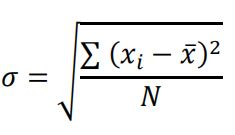5. What is the difference between long format and wide format data?

A dataset can be written in two different formats: wide and long.

📍 Wide format is where we have a single row for every data point with multiple columns to hold the values of various attributes.

📍 The long format is where for each data point we have as many rows as the number of attributes and each row contains the value of a particular attribute for a given data point.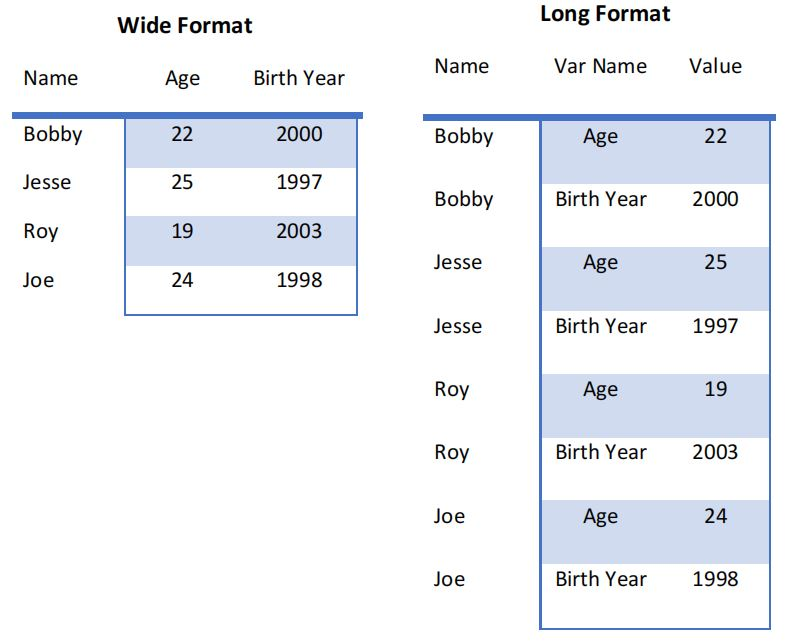6. Give an example where the median is a better measure than the mean

The median is a better measure of central tendency than the mean when the distribution of data values is skewed or when there are clear outliers.

7. How do you calculate the needed sample size?

To calculate the sample size needed for a survey or experiment:

📍 Define the population size: The first thing is to determine the total number of your target demographic. If you are dealing with a larger population, you can approximate the total population between several educated guesses.

📍 Decide on a margin of error: Also known as a “confidence interval”. The margin of error indicates how much of a difference you are willing to allow between your sample mean and the mean of the population.

📍 Choose a confidence level: Your confidence level indicates how assured you are that the actual mean will fall within your chosen margin of error. The most common confidence levels are 90%, 95%, and 99%. Your specified confidence level corresponds with a z-score.

`Z-scores for the three most common confidence levels are:`
• `90% = 1.645`
• `95% = 1.96`
• `99% = 2.576`

📍 Pick a standard of deviation: Next, you will need to determine your standard of deviation, or the level of variance you expect to see in the information gathered. If you don’t know how much variance to expect, a standard deviation of 0.5 is typically a safe choice that will ensure your sample size is large enough.

📍 Calculate your sample size: Finally, you can use these values to calculate the sample size. You can do this by using the formula or by using a sample size using a calculator online.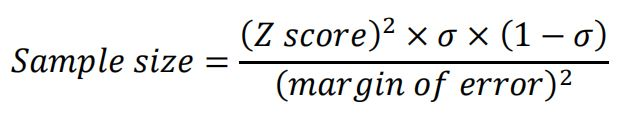8. What are the types of sampling in Statistics?

The four main types of data sampling in Statistics are:

📍 Simple random sampling: This method involves pure random division. Each individual has the same probability of being chosen to be a part of the sample.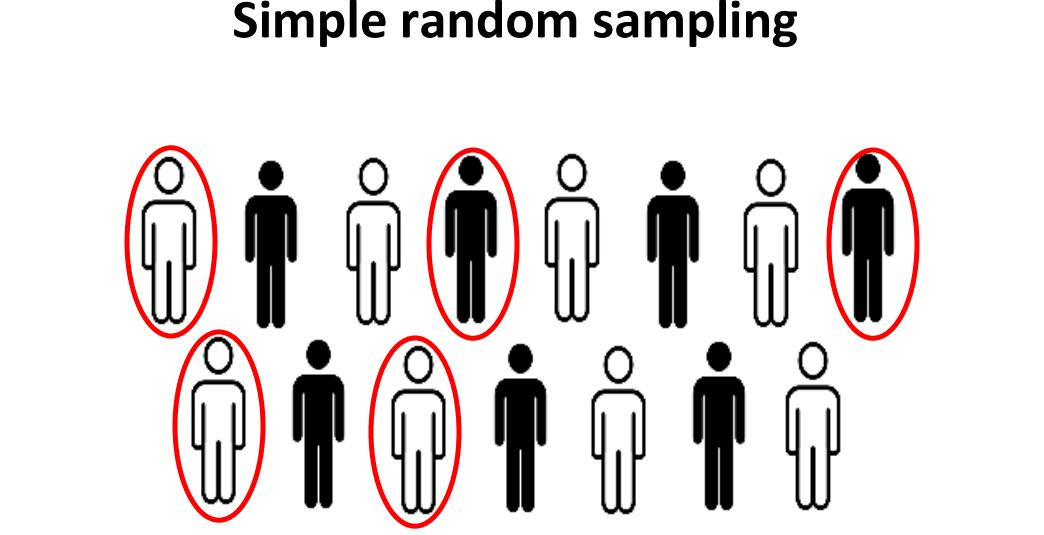📍 Cluster sampling: This method involves dividing the entire population into clusters. Clusters are identified and included in the sample based on demographic parameters like sex, age and location.

📍 Stratified sampling: This method involves dividing the population into unique groups that represent the entire population. While sampling, these groups can be organized and then drawn a sample from each group separately.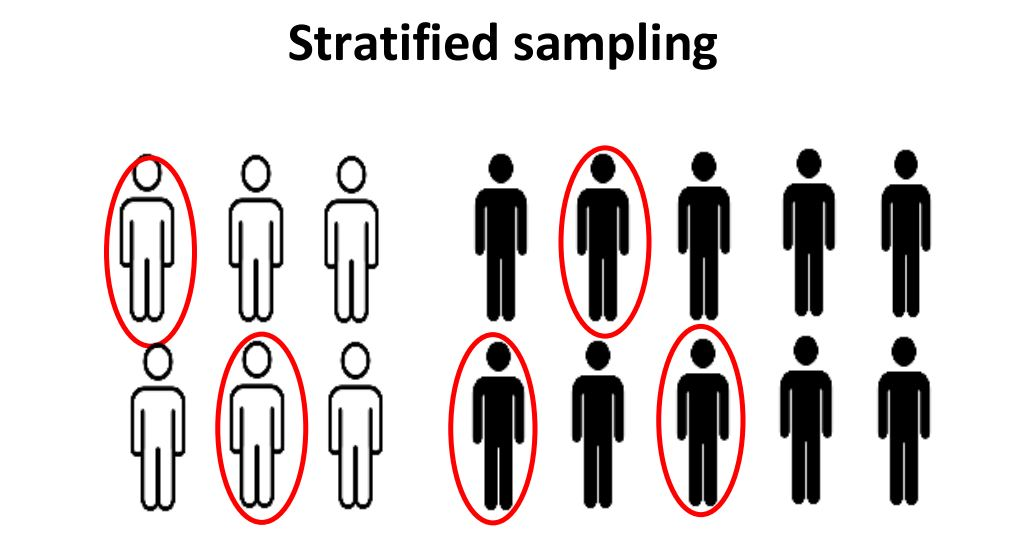📍 Systematic sampling: This sampling method involves choosing the sample members from a larger according to a random starting point but with a fixed, periodic interval called sampling interval. The sampling interval is calculated by diving the population by the desired sample size. This type of sampling method has a predefined range, hence the least time-consuming.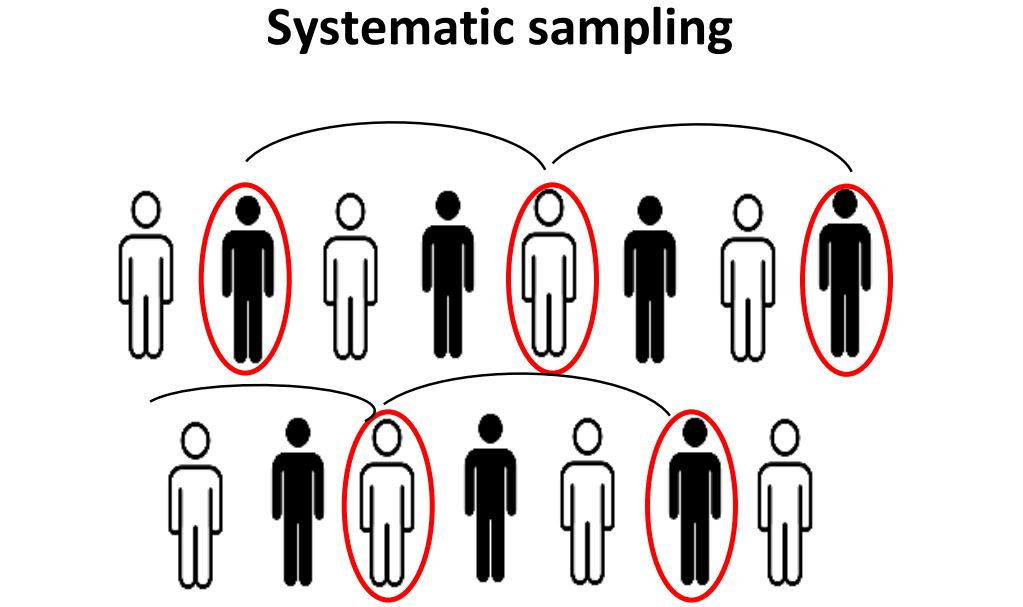9. What is Bessel’s correction?

In statistics, Bessel’s correction is the use of n-1 instead of n in several formulas, including the sample variance and standard deviation, where n is the number of observations in a sample. This method corrects the bias in the estimation of the population variance. It also partially corrects the bias in the estimation of the population standard deviation, thereby, providing more accurate results.

10. What do you understand by the term Normal Distribution?

Normal distribution, also known as the Gaussian distribution, is a bell-shaped frequency distribution curve. Most of the data values in a normal distribution tend to cluster around the mean.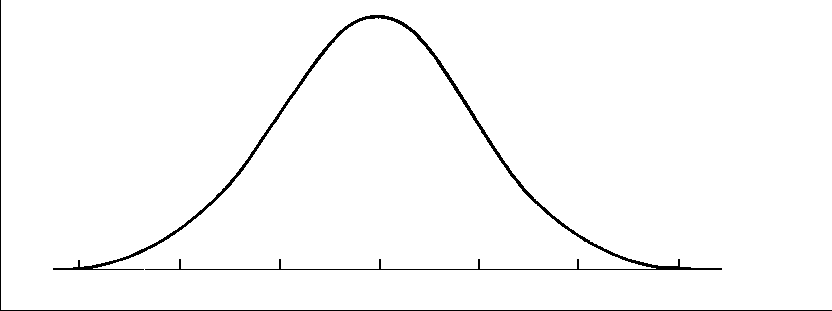11. What is the assumption of normality?

This assumption of normality dictates that if many independent random samples are collected from a population and some value of interest (like the sample mean) is calculated, and then a histogram is created to visualize the distribution of sample means, a normal distribution should be observed.

12. How do you convert a normal distribution to standard normal distribution?

The standard normal distribution, also called the z-distribution, is a special normal distribution where the mean is equal to 0 and the standard deviation is equal to 1.

Any nonstandard normal distribution can be standardized by transforming each data value x into a z-score.

To convert the point x from a normal distribution into a z-score with this formula:

`z = (x-µ) / σ`

13. What are left-skewed distribution and right-skewed distribution?

Skewness is a way to describe the symmetry of a distribution.

📍 A left-skewed (Negative Skew) distribution is one in which the left tail is longer than that of the right tail. For this distribution, mean < median < mode.

📍 Similarly, right-skewed (Positively Skew) distribution is one in which the right tail is longer than the left one. For this distribution, mean > median > mode.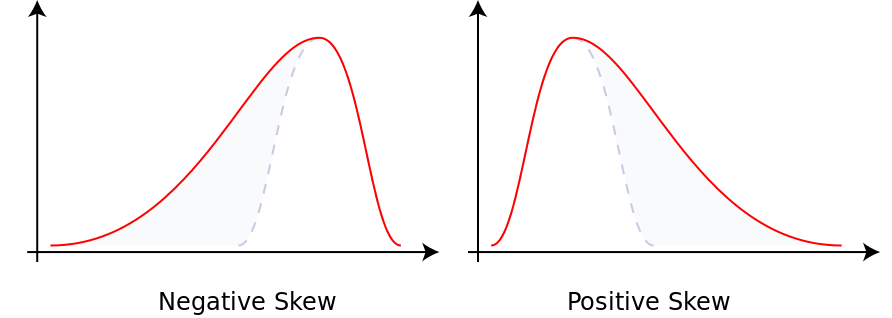14. What are some of the properties of a normal distribution?

Some of the properties of a Normal Distribution are as follows:

📍 Unimodal: normal distribution has only one peak. (i.e., one mode)

📍Symmetric: a normal distribution is perfectly symmetrical around its centre. (i.e., the right side of the centre is a mirror image of the left side)

📍 The Mean, Mode, and Median are all located in the centre (i.e., are all equal)

📍Asymptotic: normal distributions are continuous and have tails that are asymptotic. The curve approaches the x-axis, but it never touches.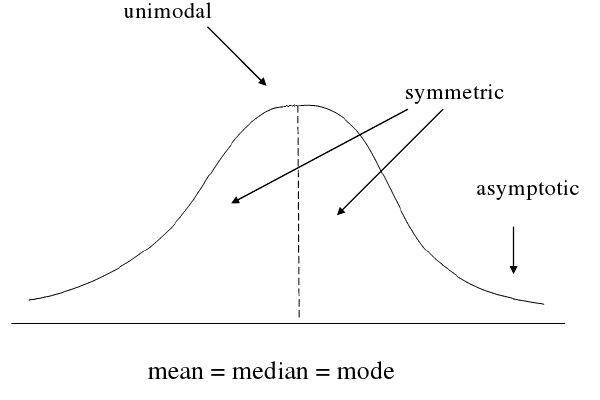15. What is the Binomial Distribution formula?

The binomial distribution formula is for any random variable X, given by;

```P(x; n, p) = nCx * px (1 – p)n – x

Where:```
• `n = the number of trials`
• `x = 0, 1, 2, ...`
• `p = probability of success on an individual trial`
• `q = 1 - p = probability of failure on an individual trial`

16. What are the criteria that Binomial distributions must meet?

The 4 criteria that Binomial distributions must meet are:

📍 There is a fixed number of trials.

📍 The outcome of each trial is independent of one another.

📍 Each trail represents one of two outcomes (“success” or “failure”).

📍 The probability of “success” p is the same across all trials.

17. What is an Outlier?

An outlier is a data point that differs significantly from other data points in a dataset. An outlier may be due to variability in measurement, or it may indicate an experimental error.

Outliers can greatly impact the statistical analyses and skew the results of any hypothesis tests.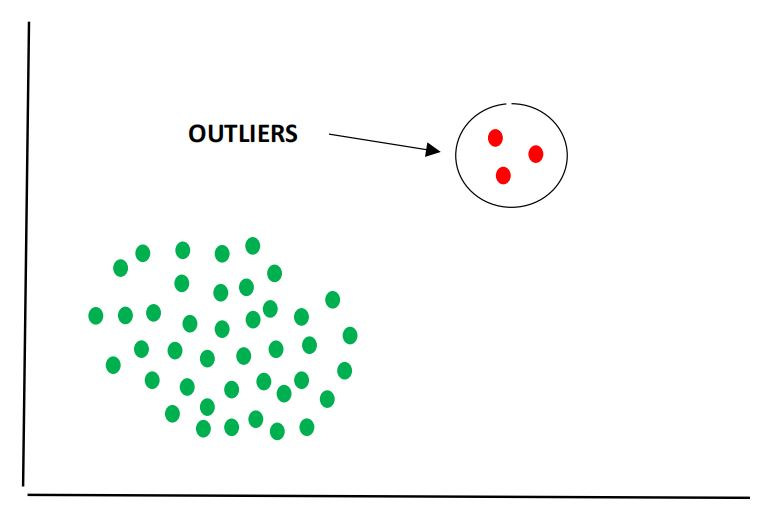It is important to carefully identify potential outliers in the dataset and appropriately deal with them for accurate results.

18. Mention methods to screen for outliers in a dataset.

📍 A simple way to check whether there is a need to investigate certain data points before using more sophisticated methods is the sorting method.

Values in the data can be sorted from low to high and then scanned for extremely low or extremely high values.

📍 Visualization (e.g. box plot) is a useful way to see the data distribution at a glance and to detect outliers. This chart highlights statistical information like minimum and maximum values (the range), the median, and the interquartile range for the data. When reviewing a box plot, an outlier is a data point outside the box plot’s whiskers.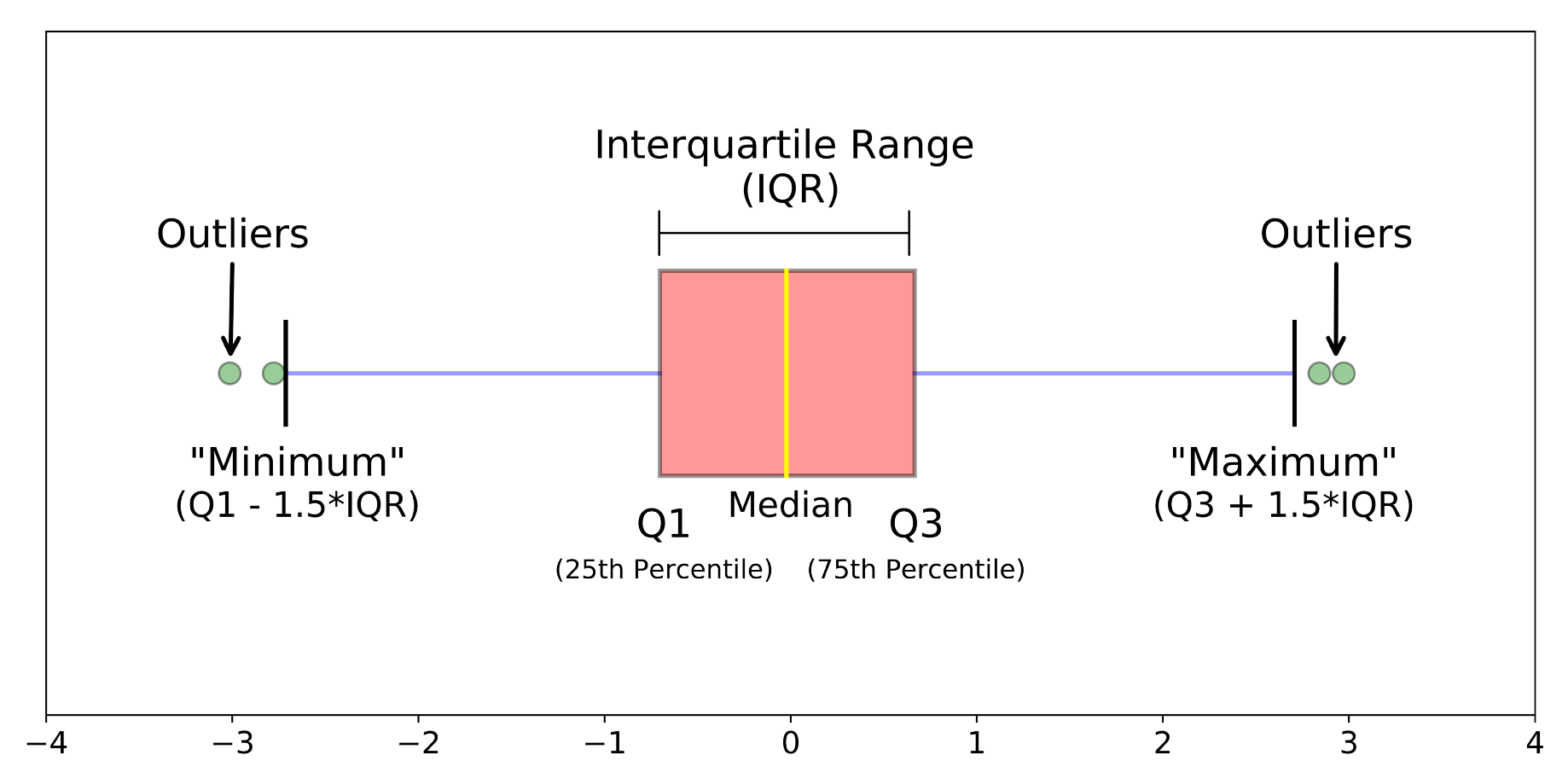📍A common method is the Interquartile range method. This method is helpful if there are few values on the extreme ends of the dataset, but you aren’t sure whether any of them might count as outliers.

The interquartile range (IQR) also called midspread tells the range of the middle half of a dataset. The IQR can be used to create “fences” around the data then, the outliers can be defined as any values greater than the upper fence or less than the lower fence.

To use the IQR method:

1. `Sort the data from low to high`
2. `Identify the first quartile (Q1), the median, and the third quartile (Q3).`
3. `Calculate the IQR; IQR = Q3 – Q1`
4. `Calculate the upper fence; Q3 + (1.5 * IQR) and the lower fence; Q1 – (1.5 * IQR)`
5. `Use the fences to highlight any outliers (all values that fall outside your fences).`

📍 Another way to identify outliers is to use Z-score. The Z-score is just how many standard deviations away from the mean value that a certain data point is. To calculate z-score use the formula, z = (x-µ) / σ

• If the z-score is positive, the data point is above average.
• If the z-score is negative, the data point is below average.
• If the z-score is close to zero, the data point is close to average.
• If the z-score is above or below 3 (assuming z-score = 3 is considered as a cut-off value to set the limit), it is an outlier and the data point is considered unusual.

Other methods to screen outliers include Isolation Forest and DBScan clustering.

19. What types of biases can you encounter while sampling?

Sampling bias occurs when a sample is not representative of a target population during an investigation or a survey. The three main that one can encounter while sampling is:

📍Selection bias: It involves the selection of individual or grouped data in a way that is not random.

📍Undercoverage bias: This type of bias occurs when some population members are inadequately represented in the sample.

📍Survivorship bias occurs when a sample concentrates on the ‘surviving’ or existing observations and ignores those that have already ceased to exist. This can lead to wrong conclusions in numerous different means.

20. What is the meaning of an inliner?

An inlier is a data value that lies within the general distribution of other observed values but is an error. Inliers are difficult to distinguish from good data values, therefore, they are sometimes difficult to find and correct.

An example of an inlier might be a value recorded in the wrong units.

21. What is hypothesis testing?

Hypothesis testing is a type of statistical inference that uses data from a sample to conclude about the population data.

Before performing the testing, an assumption is made about the population parameter. This assumption is called the null hypothesis and is denoted by H0. An alternative hypothesis (denoted Ha), which is the logical opposite of the null hypothesis, is then defined.

The hypothesis testing procedure involves using sample data to determine whether or not H0 should be rejected. The acceptance of the alternative hypothesis (Ha) follows the rejection of the null hypothesis (H0).

22. What is the p-value in hypothesis testing?

A p-value is a number that describes the probability of finding the observed or more extreme results when the null hypothesis (H0) is True.

P-values are used in hypothesis testing to help decide whether to reject the null hypothesis or not. The smaller the p-value, the stronger the evidence that you should reject the null hypothesis.

23. When should you use a t-test vs. a z-test?

📍A T-test asks whether a difference between the means of two groups is unlikely to have occurred because of random chance. It is usually used when dealing with problems with a limited sample size (n < 30).

If the population standard deviation is known, the sample size is less than or equal to 30, or if the population standard deviation is unknown, use the T-test.

📍A Z-test, on the other hand, compares a sample to a defined population and is typically used for dealing with problems relating to large samples (i.e., n > 30).

Generally, you should use a Z-test when the population’s standard deviation is known, and the sample size exceeds 30.

24. What is the difference between one-tail and two-tail hypothesis testing?

📍 One-tailed tests allow for the possibility of an effect in one direction. Here, the critical region lies only on one tail.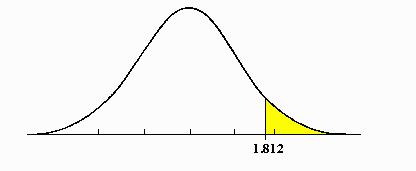📍Two-tailed tests test for the possibility of an effect in two directions—positive and negative. Here, the critical region is one of both tails.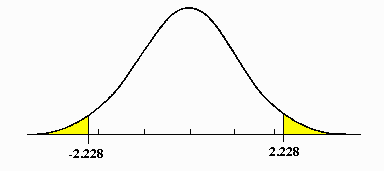25. What is the difference between type I vs. type II errors?

📍 A type I error occurs when the null hypothesis true in the population is rejected. It is also known as false-positive.

📍 A type II error occurs when the null hypothesis that is false in the population fails to get rejected. It is also known as a false-negative.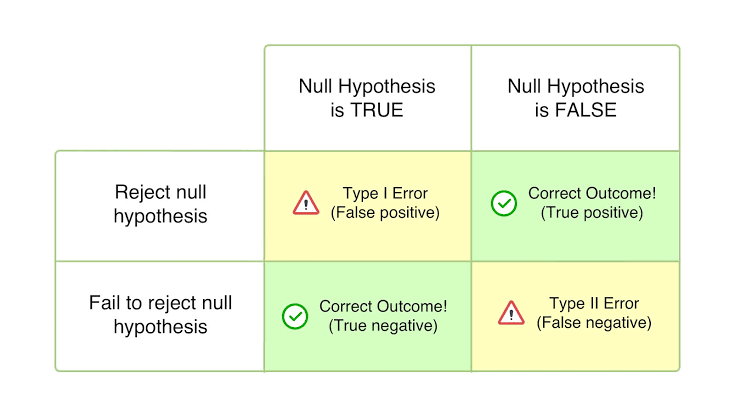26. What is the Central Limit Theorem?

The Central Limit Theorem (CLT) states that, given a sufficiently large sample size from a population with a finite level of variance, the sampling distribution of the mean will be normally distributed regardless of if the population is normally distributed.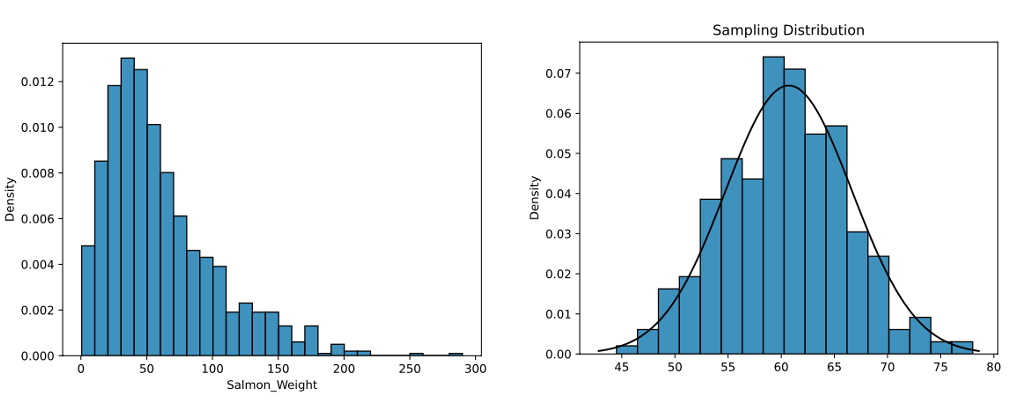27. What general conditions must be satisfied for the central limit theorem to hold?

The central limit theorem states that the sampling distribution of the mean will always follow a normal distribution under the following conditions:

📍 The sample size is sufficiently large (i.e., the sample size is n ≥ 30).

📍 The samples are independent and identically distributed random variables.

📍 The population’s distribution has finite variance.

28. What are correlation and covariance in statistics?

📍 Correlation indicates how strongly two variables are related. The value of correlation between two variables ranges from -1 to +1.

The -1 value represents a high negative correlation, i.e., if the value in one variable increases, then the value in the other variable will decrease. Similarly, +1 means a positive correlation, i.e., an increase in one variable leads to an increase in the other.

Whereas 0 means there is no correlation.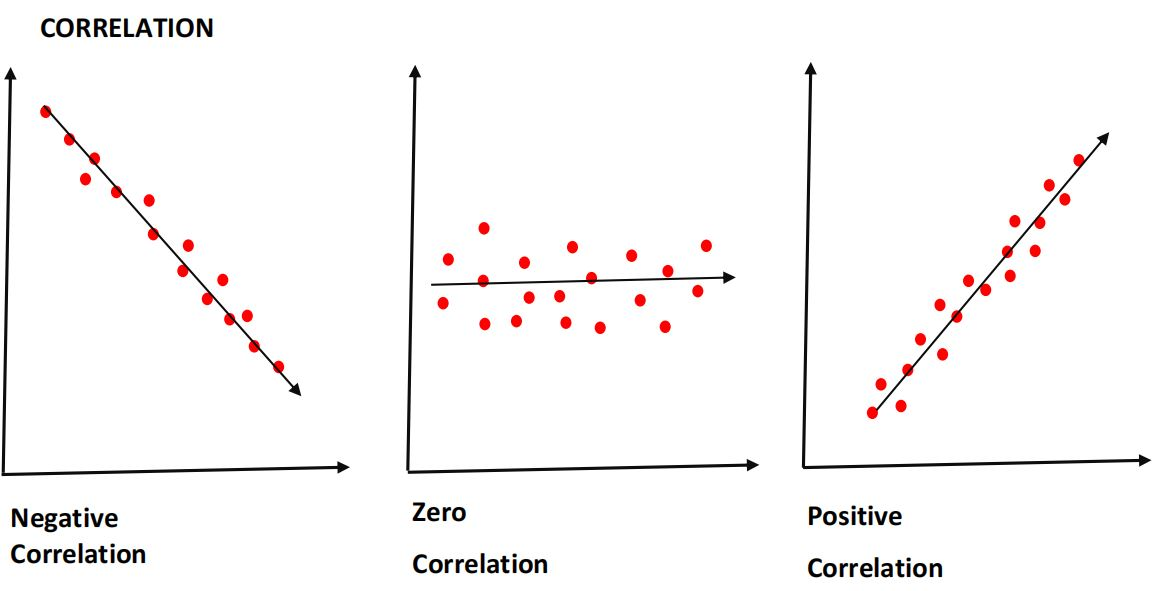📍 Covariance, on the other hand, is a measure that indicates the extent to which a pair of random variables vary with each other. A higher number denotes a higher dependency.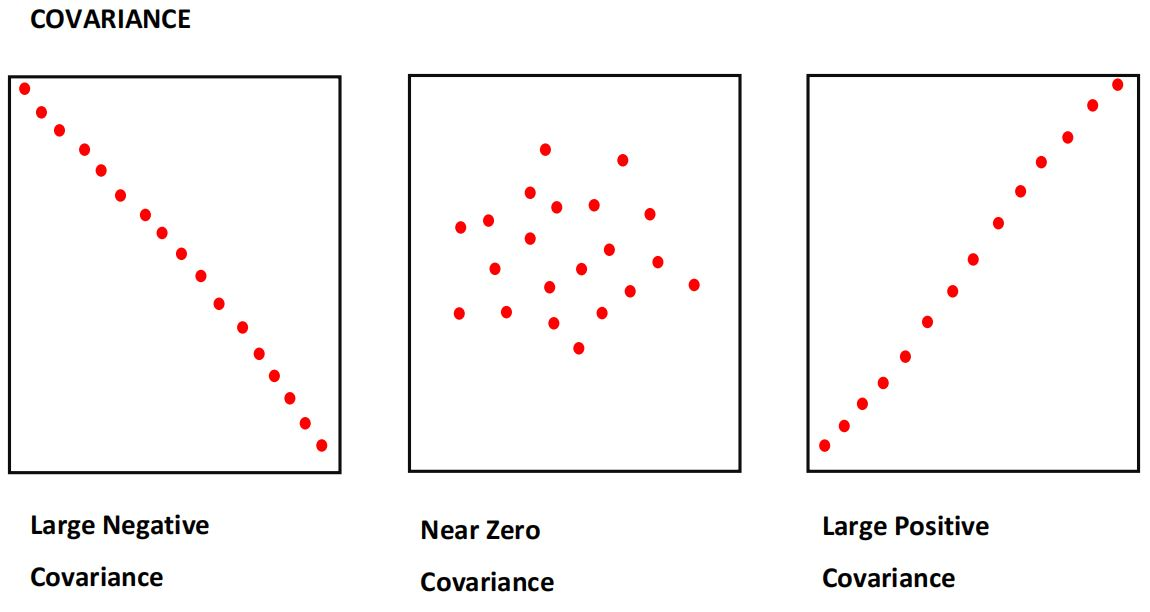29. What is the difference between Point Estimate and Confidence Interval Estimate?

📍 A point estimate gives a single value as an estimate of a population parameter. For example, a sample standard deviation is a point estimate of a population’s standard deviation.

📍 A confidence interval estimate gives a range of values likely to contain the population parameter. It is the most common type of interval estimate because it tells us how likely this interval is to contain the population parameter.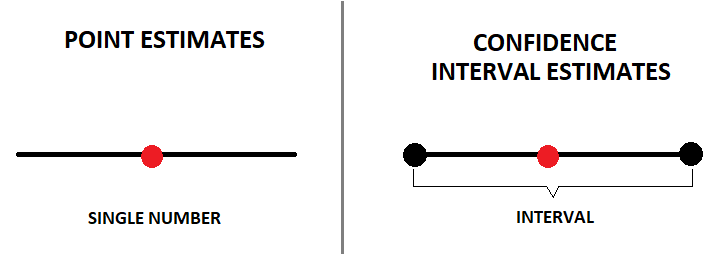30. Mention the relationship between standard error and margin of error?

As the standard error increases, the margin of error also increases.

The margin of error can be calculated using the standard error with this formula:

Margin of error = Critical value * Standard error of the sample

31. How would you define Kurtosis?

Kurtosis is the extent to which the values of a distribution’s tails differ from the centre of the distribution.

Outliers are detected in a data distribution using kurtosis. The higher the kurtosis, the higher the number of outliers in the data.

32. What is the proportion of confidence interval that will not contain the population parameter?

Alpha (α) is the portion of the confidence interval that will not contain the population parameter.

α = 1 – CL = the probability a confidence interval will not include the population parameter.

1 – α = CL = the probability the population parameter will be in the interval

For example, if the confidence level (CL) is 95%, then, α = 1 – 0.95, or α = 0.05.

33. What is the Law of Large Numbers in statistics?

According to the law of large numbers in statistics, an increase in the number of trials performed will cause a positive proportional increase in the average of the results, becoming the expected value.

For example, the probability of flipping a fair coin and landing heads is closer to 0.5 when flipped 100, 000 times compared to when flipped 50 times.

34. What is the goal of A/B testing?

A/B testing is statistical hypothesis testing. It is an analytical method for making decisions that estimate population parameters based on sample statistics.

The goal is usually to identify any changes to a web page to maximize or increase the outcome of interest. A/B testing is a fantastic method to figure out the best online promotional and marketing strategies for your business.

35. What do you understand by sensitivity and specificity?

📍Sensitivity is a measure of the proportion of actual positive cases that got predicted as positive (or true positive).

📍Specificity is a measure of the proportion of actual negative cases that got predicted as negative (or true negative).

The calculation of Sensitivity and Specificity is pretty straightforward;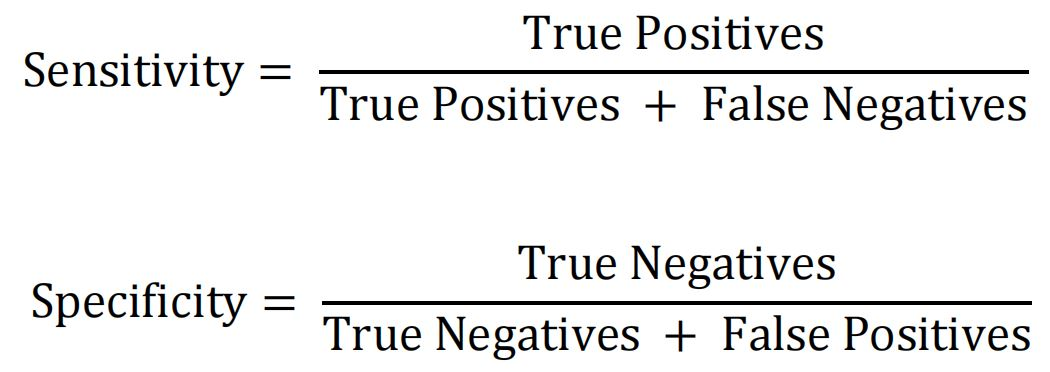36. What is Resampling and what are the common methods of resampling?

Resampling involves the selection of randomized cases with replacement from the original data sample in such a way that each number of the sample drawn has several cases that are similar to the original data sample.

Two common methods of resampling are:

1. Bootstrapping and Normal resampling
2. Cross Validation

37. What is Linear Regression?

In statistics, linear regression is an approach for modeling the relationship between one or more predictor variables (X) and one outcome variable (y).

If there is one predictor variable, it is called simple linear regression. If there is more than one predictor variable, it is called multiple linear regression.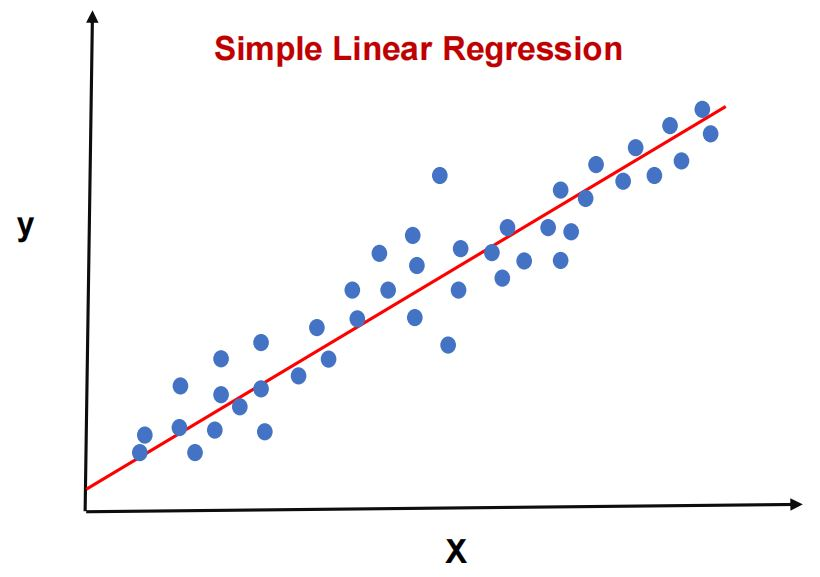38. What are the assumptions required for linear regression?

The linear regression has four key assumptions:

📍 Linear relationship: There’s a linear relationship between X and the mean of Y.

📍 Independence: Observations are independent of each other.

📍 Normality: The distribution of Y along X should be the Normal Distribution.

📍 Homoscedasticity: The variation in the outcome or response variable is the same for any value of X.

39. What is a ROC curve?

The Receiver Operator Characteristic (ROC) curve is a graphical representation of the performance of a classification model at various thresholds.

The curve plots True Positive Rate (TPR) vs. False Positive Rate(FPR) at different classification thresholds.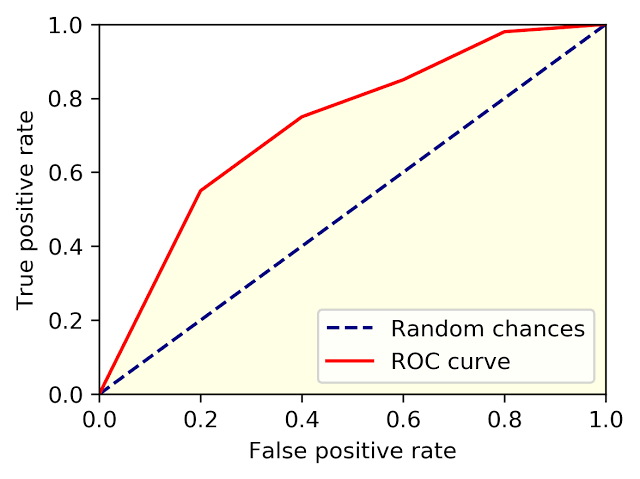40. What is Cost Function?

The cost function is an important parameter that measures the performance of a machine learning model for a given dataset.

It measures how wrong the model is in estimating the relationship between input and output parameters.

## Conclusion

This article discussed why a Data Scientist should master Statistics and some important and frequently asked Statistics Data Science Interview Questions and  Answers.

To sum up, the following are the major takeaways from the article:

• We learned about Sampling, the different types of Sampling, and how to calculate the needed sample size.
• We covered Central Tendency and Probability Distributions.
• We discussed Relationship between Variables and the difference between Covariance and Correlation.
• We covered Hypotheses Testing and P-value and discussed when to use the T-test and Z-test.
• We discussed Regression and the assumptions of Linear Regression.